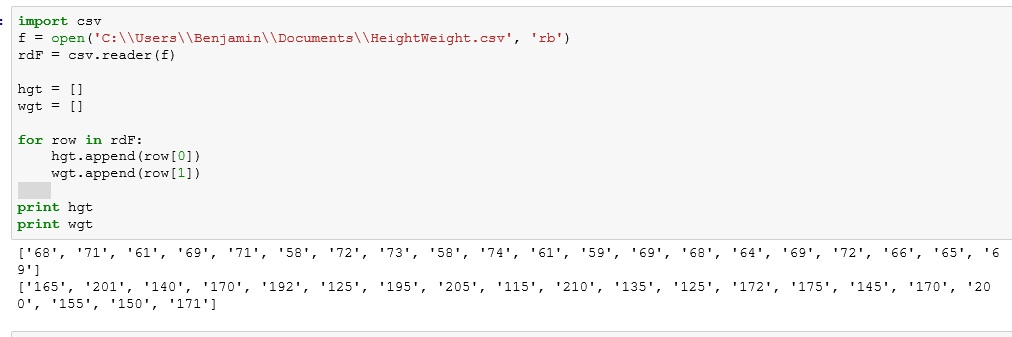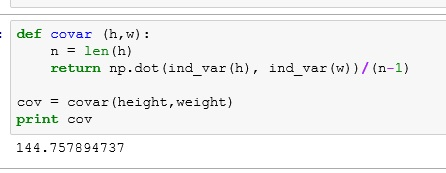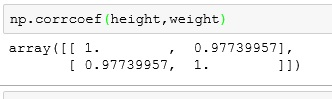# Python: Co-variance and Correlation

In this lesson, I will using data from a CSV file. You can download the file here: heightWeight

If you do not know how to import CSV files into Python, check out my lesson on it first: Python: Working with CSV Files

## The Data

The data set includes 20 heights (inches) and weights(pounds). Given what you already know, you could tell me the average height and average weight. You could tell me medians, variances and standard deviations.But all of those measurements are only concerned with a single variable. What if I want to see how height interacts with weight? Does weight increase as height increases?

**Note while there are plenty of fat short people and overly skinny tall people, when you look at the population at large, taller people will tend to weigh more than shorter people. This generalization of information is very common as it gives you a big picture view and is not easily skewed by outliers.

## Populate Python with Data

The first thing we are going to focus on is co-variance. Let’s start by getting our data in Python.Now there is a small problem. Our lists are filled with strings, not numbers. We can’t do calculations on strings.

We can fix this by populating converting the values using int(). Below I created 2 new lists (height and weight), created a for loop counting up to number of values in our lists : range(len(hgt)). Then I filled the new lists using lst.append(int(value))**Now I know I could have resolved this in fewer steps, but this is a tutorial, so I want to provide more of a walk through.

## Co-variance

Co-variance tells us how much two variables disperse from the mean together. There are multiple ways to find co-variance, but for me, using a dot product approach has always been the simplest.

For those unfamiliar with dot product. Imagine I had 2 lists (a,b) with 4 elements each. The dot product with calculated as so: a*b+a*b+a*b+a*b

Here is how it works:

If I take the individual variance of height and weight and they are both positive or negative – the product will be positive. – both variables are moving in the same direction

One positive and one negative will be negative. The variables are moving in different directions

One you add them all up, a positive number will mean that overall, you variables seem to have a positive co-variance (if a goes up, b goes up – if a goes down, b goes down)

If the final result is negative, you have negative co-variance (if a goes up, b goes down – if a goes down, b goes up)

Okay, let’s program this thing

** we will be using numpy’s mean() – mean and dot() – dot product methods and corrcoef() – correlation coefficient

First we need to find the individual variances from mean for each list

I create a function called ind_var that uses a list comprehension to subtract the mean from each element in the list.Now, let’s change out the print statement for a return, because we are going to be using this function inside another function.## Co-variance function

Now let’s build the co-variance function. Here we are taking the dot product of the variances of each element of height and weight. We then divide the result by the N-1 (the number of elements – 1 : the minus 1 is due to the fact we are dealing with sample data not population)So what were are doing:

• Take the first height (68 inches) and subtract the mean (66.8) from it (1.2)
• Take the first weight (165 lbs) and subtract the mean(165.8)  from it (-0.8)
• We then multiply these values together (-0.96)
• We repeat this for each element
• Divide by 19 (20-1)

Our result is 144.75789  – a positive co-variance. So when height goes up – weight goes up, when height goes down – weight goes down.

But what does 144 mean? Not much unfortunately. The co-variance doesn’t relate any information as to what units we are working with. 144 miles is a long way, 144 cm not so much.

## Correlation

So we have another measurement known as correlation. A very basic correlation equation divides out the standard deviation of both height and weight. The result of a correlation is between 1 and -1. With -1 being perfect anti-correlation and 1 being perfect correlation. 0 mean no correlation exists.

With my equation get 1.028  – more than one. This equation is simplistic and prone to some error.numpy’s corrcoef() is more accurate. It shows us a correlation matrix. Ignore the 1’s – they are part of what is known as the identity. Instead look at the other numbers = 0.97739.  That is about as close to one as you will ever get in reality. So even if my equation is off, it isn’t too far off.Now just to humor me. Create another list to play with.Let’s run this against height in my correlation functionRun these value through the more accurate corrcoef() . This will show my formula is still a bit off, but for the most part, it is not all that bad.If you enjoyed this lesson, click LIKE below, or even better, leave me a COMMENT.

1.Karan Jakhar
2.Ruksana